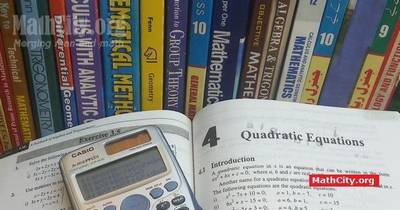Notes (Solutions) of Chapter 04: Quadratic Equations, Text Book of Algebra and Trigonometry Class XI (Mathematics FSc Part 1 or HSSC-I), Punjab Textbook Board, Lahore.

• Introduction
• Exercise 4.1
• Solutions of Equations Reducible to the Quadratic Equation
• Exercise 4.2
• Exercise 4.3
• Three Cube Roots of Unity
• Properties of Cube Roots of Unity
• Four Fourth Roots of Unity
• Properties of Four Fourth Roots of Unity
• Exercise 4.4
• Polynomial Function
• Theorems
• Synthetic Division
• Exercise 4.5
• Relations between the Roots and the Coefficients of a Quadratic Equation
• Formation of an Equation whose Roots are given
• Exercise 4.6
• Nature of the Roots of Quadratic Equation
• Exercise 4.7
• System of two Equations Involving two Variables
• Exercise 4.8
• Exercise 4.9
• Exercise 4.10

The following notes are provided by M. Shahid Nadeem, Lecturer in Mathematics, Punjab College Wah Cantt. We are very thankful to him for providing these notes.

The following notes are provided by Mr. Akhtar Abbas. A video explanation of the solutions is available at his YouTube channel Suppose Math.

• Exercise 4.1 | Download PDF• Exercise 4.2 | Download PDF• Exercise 4.3 | Download PDF• Exercise 4.4 | Download PDF• Exercise 4.5 | Download PDF• Exercise 4.6 | Download PDFThe following short questions was send by Mr. Akhtar Abbas.

• fsc/fsc_part_1_solutions/ch04